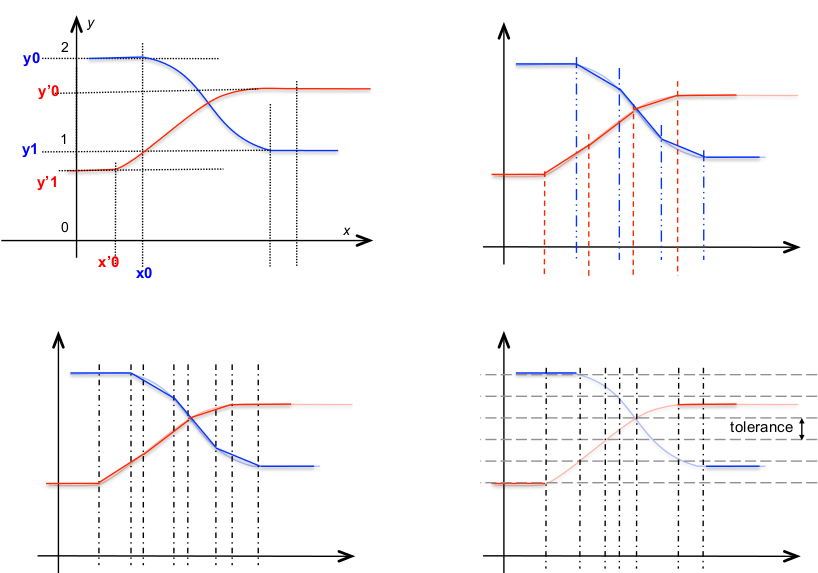@sample(nim, n:integer)


build a new nim with linear interpolation type by sampling each component with n points equally spaced between the first and the last breakpoint.

The result is a nim with linear interpolation type for all component, irrespectively of the interpolation type of the nim argument. The resulting nim can be seen as a linear approximation of the original curve.

The sampling is made component by components, so the results is not necessarily homogeneous. The function @align_breakpoints can be used on a nim with linear interpolation type to obtain an equivalent nim with homogeneous breakpoints

The approximation made with sampling on n points is not always satisfactory because the variation of the nim can differ greatly between two intervals. The function @linearize uses an adaptive sampling step to linearize the nim, to align the breakpoints and to achieve an approximation within a given tolerance .

See also @align_breakpoints, @sample and the nim simplification functions: @simplify_radial_distance_t, @simplify_radial_distance_v, @simplify_lang_v, @filter_median_t, @filter_min_t, @filter_max_t, @window_filter_t

In the figure below, the diagram at the top left shows a vectorial nim with two components:

• the effect of @sample is pictured at top right,

• the effect of @align_breakpoints is sketched at bottom left,

• and the effect of @linearize is illustrated at bottom right.